• 图的运算：删点 删边 并运算 交运算 差运算 对称差运算 联运算 积图
千次阅读
2020-03-06 20:20:41

# 1. 删点

删点要删边: 边必须要有端点才能存在；

# 2. 删边

删边不删点：点可以独立存在；

# 3. 并运算∪

G1∪G2

两个图的并: 点是点的并，边是边的并

特别地，若两个图不相交(无公共顶点)，则称它们地并为直接并，即为G1+G2;

# 4. 交运算∩

两个图的交: 点是点的交，边是边的交；

# 5. 差运算

G1-G2：从G1中删去G2中的得到的新图；

# 6. 对称差运算

G1ΔG2 = G1∪G2 - (G1∩G2)

# 7. 联运算

联运算是对两个不相交的图G1，G2而做的。

G1VG2: 先做G1+G2，之后将G1中每个顶点与G2中每个顶点连接，得到的新图即为G1VG2;

# 8. 积图

积图G1×G2，通过分别求出点集和边集得到最终的积图。

点集：V=V1×V2， 即V1与V2的笛卡尔积。例如，V1={1，2}；V2={3，4，5}，则V={(1,3),(1,4),(1,5),(2,3),(2,4),(2,5)}.

边集：若顶点u=(u1,u2),v=(v1,v2)满足如下两个条件之一，则连接uv；否则，不连接u,v.

以上便是几种主要的图的运算。

参考：

张先迪等《图论及其应用》

更多相关内容
• 博文首先贴出交集、并集、差集、对称差运算的C++代码以及程序运行结果，然后再进行一个比较完整的构建代码思路解析，整个项目的实现功能如下： 文章目录 1. 实现代码以及程序运行结果 1.1 头文件定义 UFSets.h 1.2 ...

博文首先贴出交集、并集、差集、对称差运算的C++代码以及程序运行结果，然后再进行一个比较完整的构建代码思路解析，整个项目的实现功能如下：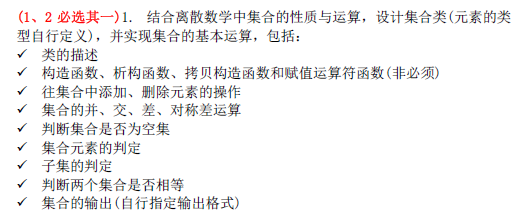# 1. 实现代码以及程序运行结果

代码不太理解的，看下面解析。

## 1.1 头文件定义 UFSets.h

//该文章的原创作者：阿伟加油鸭。首发于CSDN平台，装载请标注博文出处。
//网址：https://blog.csdn.net/qq_45877524/article/details/106972508

/**
选择题目一：
结合离散数学中集合的性质于运算，设计集合类（元素的类型自行定义),并实现集合的基本运算，
包括：
……
**/

#ifndef UFSETS_H_
#define UFSETS_H_
#include<iostream>
#include<vector>
#include<algorithm>
#include<iterator>
#include<ostream>

using namespace std;

/**

UFSets类的设计思路：
用STL的容器vector以及algorithm库的算法来进行设计

**/
class UFSets {
private:
/**

用vector的原因：
1.vector自带智能指针，不需要进行特殊的删除，所以可以用默认析构函数
2.vector本身自带了许多检测函数比较方便

**/
vector<int> data;

public:
UFSets();                   //构造函数，用来在空集上确定具体的元素
~UFSets();                  //析构函数，这里用默认构造函数

bool isEmpty();             //确定是否为空集
bool isContain(int element) const;             //确定是否包含某一个元素
bool isEquality( const UFSets& anotherSets);   //判断两个集合是否相等
bool isSubSets(const UFSets& anotherSets);    //判断是否为子集

void addElement(int element[], int length);      //增加一组元素
void del(int element);                           //删除一个元素

UFSets unionSet(const UFSets& anotherSets);          //并集操作
UFSets intersectSet(const UFSets& anotherSets);      //交集操作
UFSets differenceSet(const UFSets& anotherSets);      //差集操作
UFSets symDif(const UFSets& anotherSets);            //全名：Symmetric difference，对称差运算

int getCount() const;           //返回元素的个数
void Print() const;             //打印当前的元素

};

#endif


## 1.2 抽象基类的实现 UFSets.cpp

//该文章的原创作者：阿伟加油鸭。首发于CSDN平台，装载请标注博文出处。
//网址：https://blog.csdn.net/qq_45877524/article/details/106972508

#include "UFSets.h"

//首先将vector容器设置为空
UFSets::UFSets()
{
data = { };
}

UFSets::~UFSets()
{
}

bool UFSets::isEmpty()
{
//bool std::vector<int>::empty() const 如果为空返回true，不为空为false
if (data.empty() == true)
return true;
else
return false;
}

bool UFSets::isContain(int element) const
{
//find函数用迭代器找元素，找的到就返回他在vector的位置，找不到就算球
if (find(data.begin(), data.end(), element) != data.end())
return true;
else
return false;
}

//其实该函数可以用vector自带的重载运算符==，来进行判断，但是打完才想起来算了
bool UFSets::isEquality(const UFSets& anotherSets)
{
if (data.size() != anotherSets.data.size()) {        //连长度都不相等，肯定不相等
return false;
}

for (int i = 0; i < data.size(); i++) {
for(int j = 0;j < anotherSets.data.size();j++)   //一个一个元素进行比较
if (data[i] != anotherSets.data[j])
return false;
}

return true;
}

//algorithm函数库中的includes函数来判断子集
bool UFSets::isSubSets(const UFSets& anotherSets)
{
return includes(data.begin(), data.end(), anotherSets.data.begin(), anotherSets.data.end());
}

//vector语法
{
data.push_back(element);              //push_back函数为容器后面添加元素
}

//vector语法
{
for (int i = 0; i < length; i++)

sort(data.begin(),data.end());
}

void UFSets::del(int element)
{
if (isContain(element) == false) {
cout << "没有这个元素删除个蛇皮" << endl;                   //都不在删除个蛇蛇皮
return ;
}

auto location = find(data.begin(), data.end(), element);        //找到特定元素，然后直接删除

data.erase(location);
}

/**
交、差、并、对称差运算都是用到了algorithm函数库中的函数
这四个函数的语法都是通用的：

以set_union为例：
set_union(data.begin(),data.end(),anotherSets.data.begin(),anotherSets.data.end(),
inserter(result.data,result.data.begin()));

前面四个参数都是vector的迭代器，最后一个参数是输出，那么这里在输出那里用inserter函数，将输出的
结果直接搞到vector容器中
**/
UFSets UFSets::unionSet(const UFSets& anotherSets)
{
UFSets result;
set_union(data.begin(),data.end(),anotherSets.data.begin(),anotherSets.data.end(),
inserter(result.data,result.data.begin()));
return result;
}

UFSets UFSets::intersectSet(const UFSets& anotherSets)
{
UFSets result;
set_intersection(data.begin(), data.end(), anotherSets.data.begin(), anotherSets.data.end(),
inserter(result.data, result.data.begin()));
return result;
}

UFSets UFSets::differenceSet(const UFSets& anotherSets)
{
UFSets result;
set_difference(data.begin(), data.end(), anotherSets.data.begin(), anotherSets.data.end(),
inserter(result.data, result.data.begin()));
return result;
}

UFSets UFSets::symDif(const UFSets& anotherSets)
{
UFSets result;
set_symmetric_difference(data.begin(), data.end(), anotherSets.data.begin(), anotherSets.data.end(),
inserter(result.data, result.data.begin()));
return result;
}

//元素的个数直接用vector的函数方法
int UFSets::getCount() const
{
return data.size();
}

//打印元素
void UFSets::Print() const
{
cout << "当前集合的元素" << endl;
for (int i = 0; i < data.size(); i++) {
cout << data[i] << " "<<endl;
}
cout << "完成" << endl;
}



## 1.3 测试函数以及测试用例 main.cpp

#include "UFSets.h"
/**

测试用例：
set1 = {1 3 5 7 9}
set2 = {2 4 6 7 9 10}

set2在删除元素2后的预测结果：
set2 = {4 6 7 9 10}

用set1进行属性测试函数的验证：

预期结果：
是否为空集              不是
是否包含元素1           是
是否与set2相等          不是
set1是否为set2的子集    不是

用set1和删除元素2以后的set2进行并，交，差，对称差运算的结果预测：
并集          1 3 4 5 6 7 9 10
交集          7 9
差集          1 3 5
对称差运算    1 3 4 5 6 10

**/

int main() {
int setOne[] = { 1,3,5,7,9 };
int setTwo[] = { 2,4,6,7,9,10 };

UFSets set1, set2;

//打印这两个东西
set1.Print();
set2.Print();

//把2删除试一下
set2.del(2);
set2.Print();

//并，交，差，对称差运算
cout << "并集" << endl;
UFSets UnionSet = set1.unionSet(set2);
UnionSet.Print();

cout << "交集" << endl;
UFSets IntersectSet = set1.intersectSet(set2);
IntersectSet.Print();

cout << "差集" << endl;
UFSets DifferenceSet = set1.differenceSet(set2);
DifferenceSet.Print();

cout << "对称差运算" << endl;
UFSets symdif = set1.symDif(set2);
symdif.Print();

//返回set1的元素个数
cout << "接下来都是set1的验证" << endl;
cout << set1.getCount() << endl;
cout << "是否为空集" << " "<<set1.isEmpty() << endl;
cout << "是否包含元素" <<" " <<set1.isContain(1) << endl;
cout << "是否相等" <<" "<< set1.isEquality(set2) << endl;
cout << "set1是否为set2的子集" <<" "<< set1.isSubSets(set2) << endl;

return 0;
}


## 1.4 代码运行结果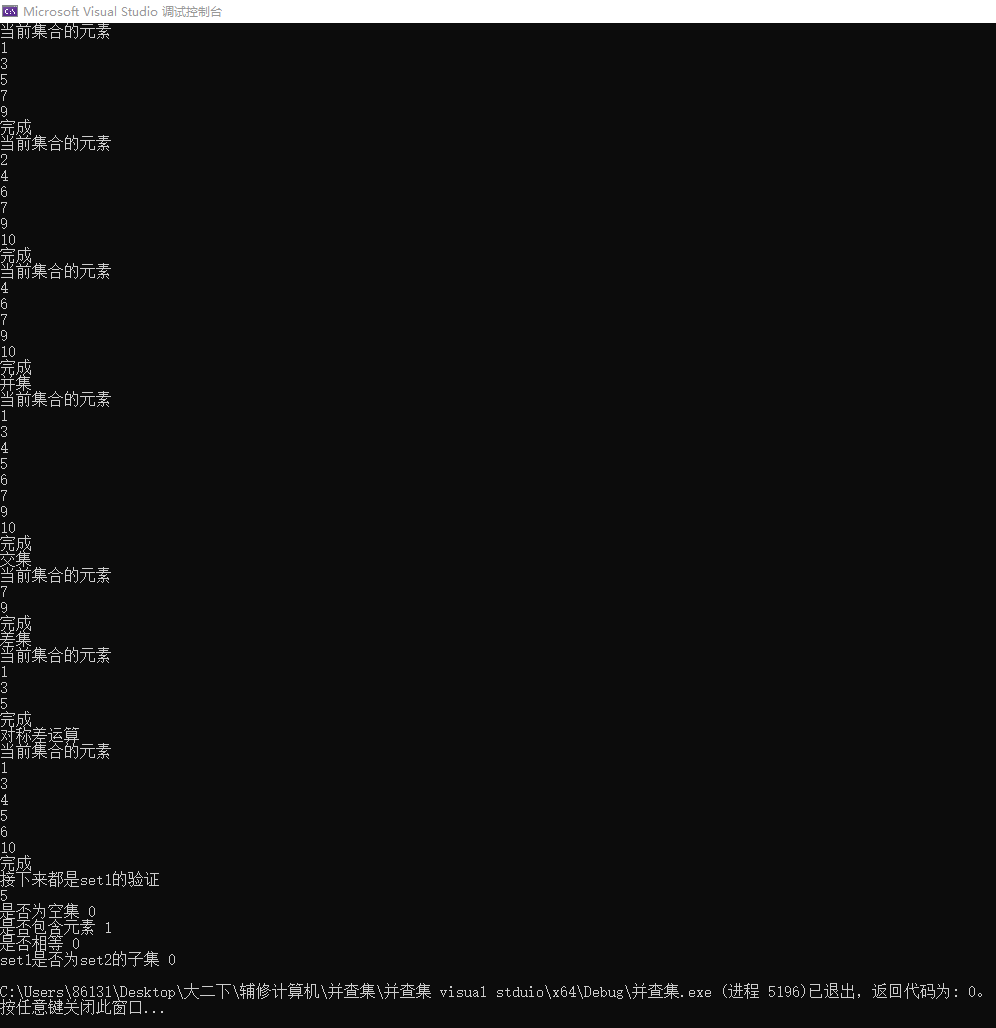## 1.5 代码环境

C++11，运行不成功可能是dev版本过老的问题，反正我用dev和visual stduio 2020都是没有问题的。

# 2 代码构建思路

我的代码构建思路可能不太成熟，望大佬指正。

设计一个类，首先应该考虑的是用什么东西来存储相应的元素，那么对于设计的需求而言，我要做的是元素（单个数据）与元素（单个数据）之间的交互。所以有两个选择，一个是数组，那么另外一个STL容器的vector（vector容器比较常用，相当于数组的2.0版本）

考虑到下面两个原因，我选择vector容器：

1. 在algorithm中本身就有差集、交集、并集和对称差运算的标准函数。它们的用法如下（看不懂的，看下面文章链接，里面有迭代器、vector容器、algorithm的介绍）：
2. vector容器可以进行自我检测，empty之类都有，可以非常方便的运算减少
当然，在交集、并集、差集、对称差运算这三个学校所谓必须要掌握，实际没个der的程序中，我也会用数组的方法，进行解释。

这是STL的介绍补充以及vector容器的用法：

1. CSDN博主禾夕的《STL vector的内部实现原理及基本用法
2. CSDN博主boke_fengwei的《c+±–vector的使用
3. CSDN博主yyce的《面向对象程序设计(C++) 示例程序——集合类
4. CSDN博主 HUST_Miao的《C++中STL用法超详细总结

# 3. 交集、并集、差集、对称差运算

有两种方法进行实现：一种是用到了algorithm函数库里面的函数，一种是直接头铁来弄。

## 3.1 运用到了algorithm函数库里面的函数

其实我这个程序，已经用到了algorithm函数库里面的函数，下面对着来讲一下：

UFSets UFSets::unionSet(const UFSets& anotherSets)
{
UFSets result;
set_union(data.begin(),data.end(),anotherSets.data.begin(),anotherSets.data.end(),
inserter(result.data,result.data.begin()));
return result;
}

UFSets UFSets::intersectSet(const UFSets& anotherSets)
{
UFSets result;
set_intersection(data.begin(), data.end(), anotherSets.data.begin(), anotherSets.data.end(),
inserter(result.data, result.data.begin()));
return result;
}

UFSets UFSets::differenceSet(const UFSets& anotherSets)
{
UFSets result;
set_difference(data.begin(), data.end(), anotherSets.data.begin(), anotherSets.data.end(),
inserter(result.data, result.data.begin()));
return result;
}

UFSets UFSets::symDif(const UFSets& anotherSets)
{
UFSets result;
set_symmetric_difference(data.begin(), data.end(), anotherSets.data.begin(), anotherSets.data.end(),
inserter(result.data, result.data.begin()));
return result;
}


那么好，对其中起主要作用的四个函数进行分析

//并集
set_union(data.begin(),data.end(),anotherSets.data.begin(),anotherSets.data.end(),
inserter(result.data,result.data.begin()));

//交集
set_intersection(data.begin(), data.end(), anotherSets.data.begin(), anotherSets.data.end(),
inserter(result.data, result.data.begin()));
//差集
set_difference(data.begin(), data.end(), anotherSets.data.begin(), anotherSets.data.end(),
inserter(result.data, result.data.begin()));

//对称差运算
set_symmetric_difference(data.begin(), data.end(), anotherSets.data.begin(), anotherSets.data.end(),
inserter(result.data, result.data.begin()));


这四个函数的注意事项以及调用方式都是一样，那么以std::set_difference为例，先说函数的调用方式，再说注意事项。

调用方式：
template<class InputIterator1,class InputIterator2,class OutputIterator>
OutputIterator set_difference(InputIterator1 first1,InputIterator1 last1,InputIterator2 first2,InputIterator2 last2,OutputIterator result)

InputIterator = 输入数组的迭代器        OutputIterator = 输出迭代器

示例：
UFSets UFSets::differenceSet(const UFSets& anotherSets)
{
UFSets result;
set_difference(data.begin(), data.end(), anotherSets.data.begin(), anotherSets.data.end(),
inserter(result.data, result.data.begin()));
return result;
}

InputIterator输入数组迭代器，直接像我这样用，这个比较明白

在调用方式这里，OutputIterator可能有点蒙，我这里推荐用inserter：

inserter(container,pos)：返回通用插入型迭代器，内部会调用容器container的insert(pos)方法将数据插入到pos位置。

用法通俗一点：

inserter(你现在用的函数，你要插入的位置)

你可以用ostream但是比较复杂一点，按着例子来吧：

set_difference(data.begin(), data.end(), anotherSets.data.begin(), anotherSets.data.end(),ostream_iterator<int>(cout," "));



//vector语法
{
for (int i = 0; i < length; i++)

sort(data.begin(),data.end());
}


四个函数非常具体的分析包括底层实现看这里：

1. CSDN博主Sim0Hayha的《【C++】关于 std::set_intersection( ) 函数用法
2. CSDN博主清远qingyuan的《C++集合操作之集合差集：std::set_difference
3. CSDN博主清远qingyuan的《C++集合操作之集合并集：std::set_union
4. CSDN博主星辰浩宇的《关于C++中使用set_union、set_intersection、set_difference，set_symmetric_difference、merge的总结

## 3.2 直接用数组

篇幅有限，看这里：CSDN博主tx何家科03的《输入两个集合求出它们的交集，并集，相对差与相对补集并判断他们是否相等

# 4. 参考资料

1. CSDN博主Sim0Hayha的《【C++】关于 std::set_intersection( ) 函数用法
2. CSDN博主禾夕的《STL vector的内部实现原理及基本用法
3. CSDN博主boke_fengwei的《c+±–vector的使用
4. CSDN博主yyce的《面向对象程序设计(C++) 示例程序——集合类
5. CSDN博主 HUST_Miao的《C++中STL用法超详细总结
6. CSDN博主清远qingyuan的《C++集合操作之集合差集：std::set_difference
展开全文c++ 算法 编程语言
• Python实现两个图的交运算、并运算、差运算、对称差运算、联运算、积图、合成图 用python随机生成两张图G1,G2 import networkx as nx import matplotlib.pyplot as plt import numpy as np from numpy import...

Python实现两个图的交运算、并运算、差运算、对称差运算、联运算、积图、合成图
用python随机生成两张图G1,G2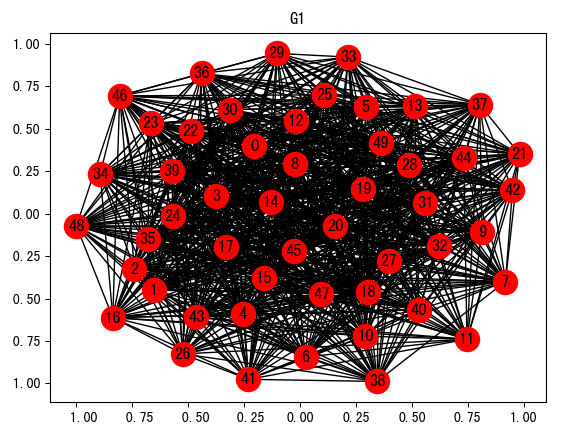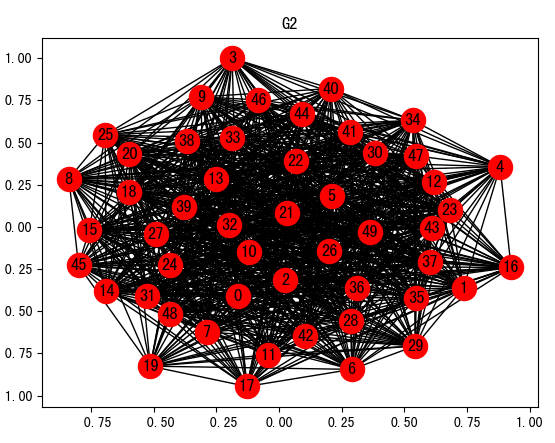import networkx as nx
import matplotlib.pyplot as plt
import numpy as np
from numpy import random

G1 = nx.Graph()
Matrix1 = np.array(random.randint((2),size=(50,50)))
#print(Matrix)
for i in range(len(Matrix1)):
for j in range(len(Matrix1)):
if Matrix1[i, j]!= 0:
nx.draw_networkx(G1)
plt.title("G1")
plt.show()

G2= nx.Graph()
Matrix2 = np.array(random.randint((2),size=(50,50)))
for i in range(len(Matrix2)):
for j in range(len(Matrix2)):
if Matrix2[i,j]!=0:
nx.draw_networkx(G2)
plt.title("G2")
plt.show()

np.union1d(Matrix1,Matrix2)
plt.title("G1与G2的并运算")
plt.show()
np.intersect1d(Matrix1,Matrix2, assume_unique=True)
plt.title("G1与G2的交运算")
plt.show()
np.setdiff1d(Matrix1,Matrix2, assume_unique=True)
plt.title("G1与G2的差运算")
plt.show()
np.setdiff1d(Matrix1,Matrix2, assume_unique=True)
plt.title("G2与G1的差运算")
plt.show()
Matrix1^Matrix2
plt.title("G1与G2的对称差运算")
plt.show()
Matrix1+Matrix2
plt.title("G1与G2的联运算")
plt.show()
Matrix1*Matrix2
plt.title("G1与G2的积运算")
plt.show()
Matrix1[Matrix2]
plt.title("G1与G2的合成运算")
plt.show()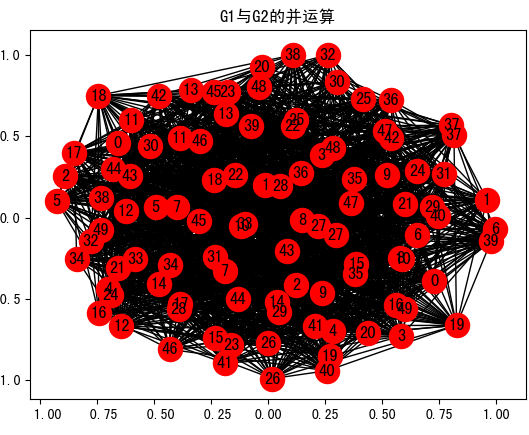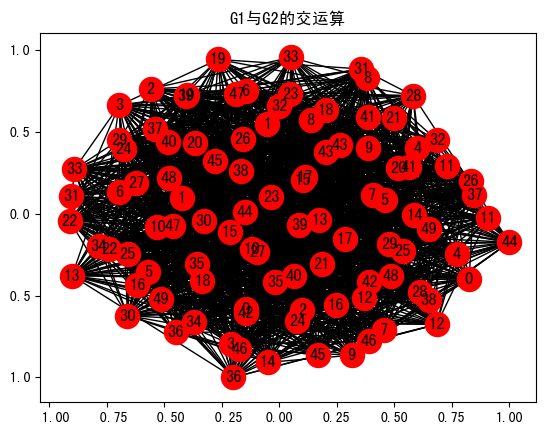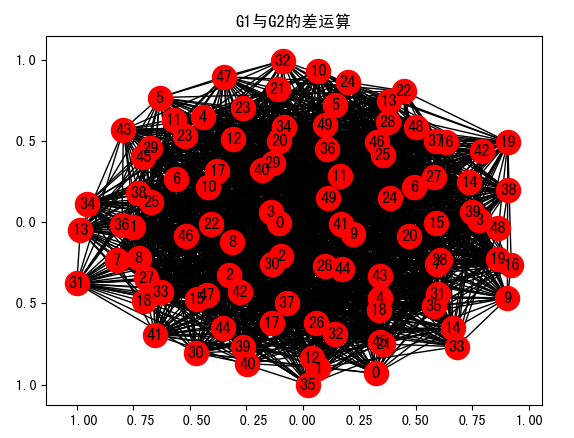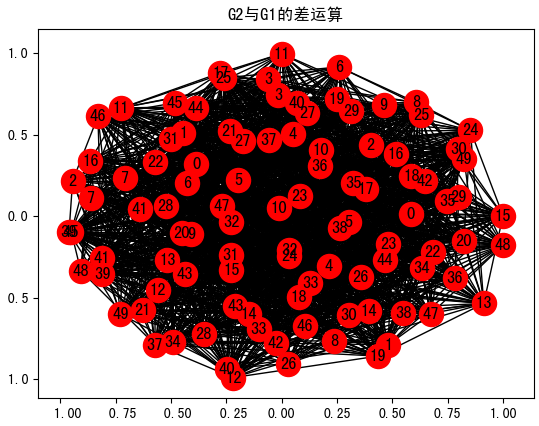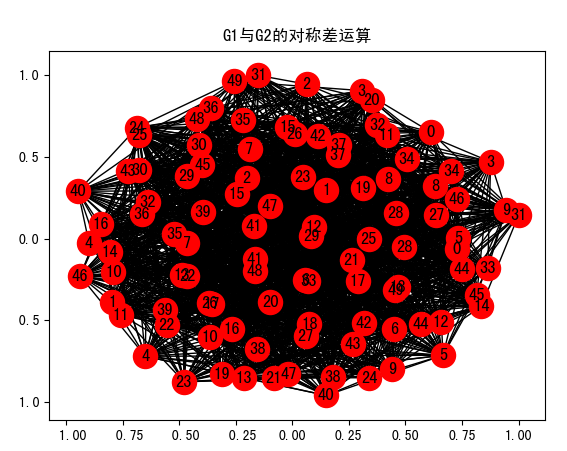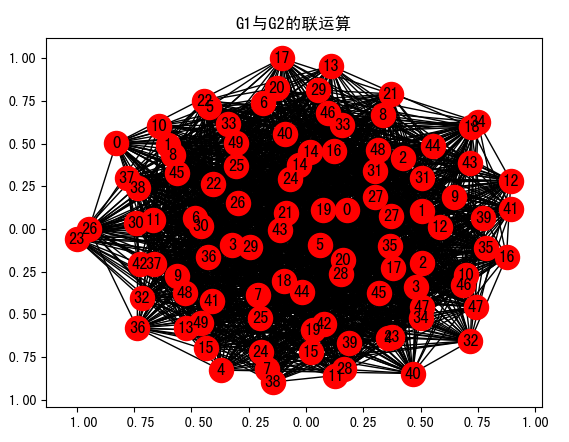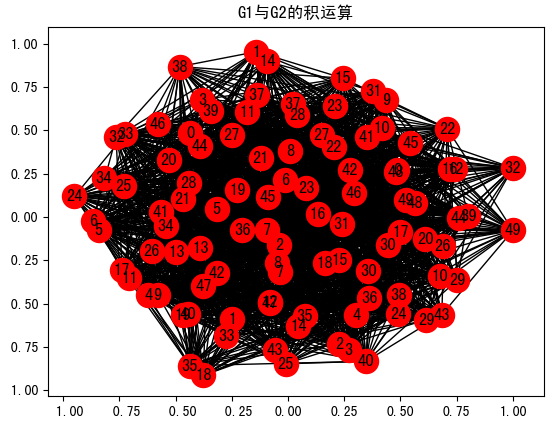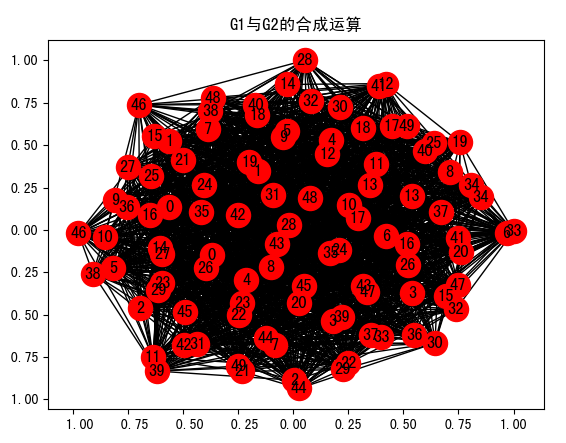展开全文• 1.集合的常用运算： 并集：X∪Y={x | x∈X或x∈Y}。 交集：X∩Y={x | x∈X且x∈Y}。 差集：X-Y={x | x∈X且x∉Y}。 如果X∩Y=∅，那么集合X与Y不相交。如果集合X和Y是集族S中的不同集合且X和Y不相交，那么集族S两两...# 关于集合的并交差

### 1.集合的常用运算：

并集：X∪Y={x | x∈X或x∈Y}。
交集：X∩Y={x | x∈X且x∈Y}。
差集：X-Y={x | x∈X且x∉Y}。
如果X∩Y=∅，那么集合X与Y不相交。如果集合X和Y是集族S中的不同集合且X和Y不相交，那么集族S两两不相交。

#include <stdio.h>
#define N 100
main()
{
int a[N],b[N],c[N],d[N],e[N],h[N];
int aa[N],bb[N],ee[N],ff[N];
int i,j,k=1;
int x=1,y=1,z=1,o=1,g=1;
int m,n,w;
int flag;
printf("请输入集合A的元素个数:\n");
scanf("%d",&m);
printf("输入集合A的元素:\n");
for(i=1;i<=m;i++)
{
scanf("%d",&a[i]);
}
printf("请输入集合B的元素个数:\n");
scanf("%c",&n);
printf("输入集合B的元素:\n");
for(i=1;i<=n;i++)
{
scanf("%d",&b[i]);
}
printf("请输入集合E(全集)的元素个数:\n");
scanf("%c",&w);
printf("输入集合E的元素:\n");
for(i=1;i<=w;i++)
{
scanf("%d",&e[i]);
}

for(i=1;i<=m;i++)//差集
{
flag=0;
for(j=1;j<=n;j++)
{
if(a[i]==b[j])
{
c[k]=a[i];
k++;
flag=1;
continue;
}
}
if(flag==0)
{
aa[x]=a[i];
x++;
}
}

for(i=1;i<=n;i++)
{
flag=0;
for(j=1;j<=m;j++)
{
if(a[j]==b[i])
{
flag=1;
continue;
}
}
if(flag==0)
{
bb[y]=b[i];y++;
}
}

for(i=1;i<=w;i++)
{
flag=0;
for(j=1;j<=m;j++)
{
if(a[j]==e[i])
{
flag=1;
continue;
}
}
if(flag==0)
{
ee[z]=e[i];z++;
}
}

for(i=1;i<=w;i++)
{
flag=0;
for(j=1;j<=n;j++)
{
if(b[j]==e[i])
{
flag=1;
continue;
}
}
if(flag==0)
{
ff[o]=e[i];o++;
}
}

for(i=1;i<=o;i++)
{
flag=0;
for(j=1;j<=z;j++)
{
if(ee[j]==ff[i])
{
flag=1;
h[g]=ff[i];
g++;
continue;
}
}

}

printf("A与B的交集={ ");
for(i=1;i<k;i++)
{
printf("%d ",c[i]);
}
printf("  }\n");

printf("A与B的并集={ ");
for(i=1;i<x;i++)
{
printf("%d ",aa[i]);
}
for(i=1;i<y;i++)
{
printf("%d ",bb[i]);
}
for(i=1;i<k;i++)
{
printf("%d ",c[i]);
}
printf("  }\n");

/*printf("A与B的环和={ ");
for(i=1;i<x;i++)
{
printf("%d ",aa[i]);
}
for(i=1;i<y;i++)
{
printf("%d ",bb[i]);
}
printf("  }\n"); */

printf("A与B的差集={ ");
for(i=1;i<x;i++)
{
printf("%d ",aa[i]);
}
printf("  }\n");

/*printf("A与B的环积={ ");
for(i=1;i<k;i++)
{
printf("%d ",c[i]);
}
for(i=1;i<g-1;i++)
{
printf("%d ",h[i]);
}
printf("  }\n"); */
}


### 2.集合的补集：所研究的集合都是某个集合U的子集，此时U称为全集或全域。给定全集U和U的一个子集X，集合U-X称为X的余（补）集。

#include<stdio.h>
#include<stdlib.h>
#include<time.h>
#define N  100
#define M 50
void create_array(int a[], int n)
{
int i;
for (i = 1; i <= n; i++) {
a[i] = ((rand() % 100) + 1);
}
a = n;
}
int cnt;
void printf_buji_arrray(int a[])
{
int i;

printf("数组为{");
printf(" ");
for (i = 1; i <= a; i++) {
printf(" %d ", a[i]);
}
printf("}\n");
}
void union_buji_array(int a[], int b[])
{
int i, j, k;
cnt = 1;
for (i = 1; i <= a; i++)
{
for (j = 1; j <= b; j++)
{
if (a[i] == b[i])
a[i] = 0;

}
}
}
int main()
{
int a[N] = { 0 }, b[N] = { 0 };
int i;
printf("随机生成数组\n");
create_array(a, 5);
printf_buji_arrray(a);
create_array(b, 5);
printf_buji_arrray(b);
union_buji_array(a, b);
printf("补集：\n");
printf("数组为{");
for (i = 1; i <= a; i++)
{
while (a[i] != 0)
{
printf(" %d ", a[i]);
break;
}
}
printf("}\n");
}



### 3.集合的环和（对称差）。给定集合A，B，定义对称差运算△如下：A△B = (A-B)∪(B-A)。

#include<stdio.h>
#include<stdlib.h>
#include<time.h>
#define N  100
#define M 50
void create_array(int a[], int n)
{
int i;
for (i = 1; i <= n; i++) {
a[i] = ((rand() % 100) + 1);
}
a = n;
}
int cnt;
void printf_huanhe_arrray(int a[])
{
int i;
printf("数组为{  ");
for (i = 1; i <= a; i++) {
printf(" %d ", a[i]);
}
printf("}\n");
}
void union_huanhe_array(int a[], int b[])
{
int x, i, j, temp;
cnt = 0;
for (i = 1; i <= a; i++)
{
x = a[i];
temp = 0;
for (j = 1; j <= b; j++)
if (x == b[j])
temp = 1;

if (temp)
{
a[i] = -10;
cnt++;

}
}

}
void bingji_huanhe_array(int a[], int b[])
{
int x, i, j;
for (i = 1; i <= b; i++)
{
x = b[i];
for (j = 1; j <= a; j++)
if (x == a[j])
break;
if (j>a) {
a[a + 1] = x;
a++;
}
}

}
int main()
{
int a[N] = { 0 }, b[N] = { 0 };
printf("随机生成数组");
create_array(a, 5);
printf_huanhe_arrray(a);
create_array(b, 5);
printf_huanhe_arrray(b);
union_huanhe_array(a, b);
union_huanhe_array(b, a);
bingji_huanhe_array(a, b);
printf("环和");
printf_huanhe_arrray(a);
}


展开全文算法 c语言 数据结构
• 主要讲的是集合运算的相关的概念，及运算方法，包括：集合的交集、集合的并集、集合的差集、集合的对称差集、集合的交集运算方法、集合的并集运算方法、集合的差集运算方法、集合的对称差运算方法。python 开发语言 后端
• } /*The difference has 3 elements: 5 15 25 */ 对称差 // set_symmetric_difference example #include // cout #include // set_symmetric_difference, std::sort #include // vector using namespace std;...
• ②输出集合A与B的并、交、相对补、差、对称差运算。 ③输出集合A与B是否相等的断定结果。 ④输出集合A与B的幂集。*/ #include "stdio.h" int disc; void xd(int a[],int b[],int n,int m) {
• 通过使用c++的类 ，容器等相关知识来实现集合间的：交 并 补 差 对称差这五种关系 这里不直接set中有关集合关系的函数 相关数学知识 并集：设A与B是任意两个集合，由至少属于集合A与集合B之一的一切元素构成的集合...c++
• ## 对称差的结合律

千次阅读 2020-11-25 20:52:20
对称差的结合律 对于集合 A,BA,BA,B，有 (A⊕B)⊕C=((A−B)∪(B−A))⊕C=((A∪B)∩(Aˉ∪Bˉ))⊕C=((A∪B)∩(Aˉ∪Bˉ)∪C)∩((Aˉ∩Bˉ)∪(A∩B)∪Cˉ)=((A∪B∪C)∩(Aˉ∪Bˉ∪C))∩(((Aˉ∪B)∩(A∪Bˉ))∪Cˉ)=...数学
• 编写程序实现两个集 合的并、交，相对补，对称差，幂集的运算并判断两个集合是否相等c语言 模块化
• 1、集合的异或运算(AΔB)定义属于A或属于B，但不同时属于A和B的元素的集合称为A和B的对称差，即A和B的异或。 ...2.2 对称差运算的交换律(AΔB)ΔC = (AΔC)ΔB 注：图1中草绿色部分为 (A...
• c语言求交、并、补、对称差 #include <stdio.h> #include <stdlib.h> #define N 50 //求并集，ab是两个集合lengtha是a的长度 void bing(float a[],int lengtha,float b[],int lengthb) { float c[N]= {0};...
• 命题逻辑异或与精确表达异或精确表达:真值表需要精确对应命题公式的简化与命题等价命题公式的...其运算法则为： a⊕b = (¬a ∧ b) ∨ (a ∧¬b) 如果a、b两个值不相同，则异或结果为1。如果a、b两个值相同，异或结果
• 一、 并集 、 二、 并集示例 、 三、 交集 、 四、 交集示例 、 五、 不相交 、 六、 相对补集 、 七、 对称差 、 八、 绝对补集 、 九、 广义并集 、 十、 广义交集 、 十一、 集合运算优先级相对补集
• 功能：能演示执行集合的并交差运算 通过有序链表来实现此次运算
• C语言入门，写的不好多多指教！//输入两个整数集合A、B，求出交集、并集以及B在A中的余集，输入时按照大小顺序输入。#include#define MAX 1000//最大输入个数int bubbleSort(int *A,int len)//冒泡排序{int i,j,t;...
• //第一个问题：编程实现集合的交、并、差运算 #include<iostream> #include<stdio.h> #include<time.h> using namespace std; void grbq(char s[]) { time_t rawtime; struct tm * timeinfo;...c++
• 总时间限制:1000ms 内存限制: 65536kB描述有两个整数集合A和B，现在要求实现集合的并、交、差运算。例如A＝{2，7，9} ，B＝{3，7，12，2}，则集合的并C＝A∪B＝{2，7，9，3，12}，而集合的交 C＝A∩B＝{2，7}，集合...
• ## 异或与对称差

千次阅读 2014-06-29 23:56:10
表示异或运算，用⊕表示两个集合的对称差运算。而在集合论里面用 △ 来表示对称差，在上学期学的数字逻辑里面⊕表示异或运算。这些一样的符号在不同科目里面表示不一样的运算，一样的运算在不同科目里面用不同的符号...数学 数据 服务器 备份
• 对序列红外图像做对称差运算,通过图像差减运算和自适应阈值分割提取目标可能的运动区域,并对区域做扩张和叠加处理,得到连续帧间目标可能出现的区域。计算每个区域红外图像的光流场,对光流场进行阈值分割,辅以数学...
• 补一下数学基础 集合间的数学操作 ... 对称差集: 调用函数symmetric_difference() 或者 ^ ，原集合不发生变化 代码 """ 集合间的数学操作 交集: 调用函数intersection（）或者 & ，原集合不发生python
• 题目：C语言实现两个集合的并、交、、补运算 环境：DEV C++ 结果图如下： 完整代码如下： #include<stdio.h> #include<stdlib.h> #define TRUE 1 #define FALSE 0 #define OK 1 #define ...
• 该算法综合帧间差分算法及背景减算法获得的信息分割运动物体, 利用对称差分算法获得中间帧运动目标的轮廓信息, 以该轮廓的外接矩形为分界, 再对其外部进行动态背景更新处理, 并对其内部进行减背景运算. 实验结果...
• ''' 已知两个列表： l1=[2,3,5,8,0] l2=[5,3,9,0] 找出两个列表的不同元素 找出两个列表的共同元素 ...#对称差集 result=(s1|s2) -(s1&s2) print(result) result=s1^s2 #在两个列表中不一样的...python
• 定义：数学上，两个集合的对称差是只属于其中一个集合，而不属于另一个集合的元素组成的集合。 集合论中的这个运算相当于布尔逻辑中的异或运算。 1.A Δ B = (A − B) ∪(B − A) 2.A Δ B = (A ∪B) − (A ∩B) ...
• 1.difference(gather_2)) - 操作符 gather_1 = {1,2,3,4,5,6,7,8,9} gather_2 = {1,2,3,5,9} print(gather_1 - gather_2) 对称差集 symmetric_difference() 和 ^ 操作符 symmetric_difference()实现集合对称差集,...python 开发语言 后端
• 掌握集合的交、并、和补运算，并且使用计算机编程实现。 二、实验内容 通过编程实现求给定集合A和B的并集C（C=A∪B）、交集C（C=A∩B）、差集C（C=A-B）、补集~C=E-C的运算。 三、实验要求 C、C＋＋或Jave...
• printf(" 并运算符：| 交运算符：& 相对补运算符：- 对称差符：+ 空集：#\n"); printf("*******************************************************************************\n"); printf("请输入集合公式："); ...集合表达式 C语言 算法
• 比如，集合1,2,3和集合3,3,4,5的对称差是集合1,2,4,5。 想到的解法，将两个排序，两个集合分别用两个工作指针i,j。比较两个指针指向的元素，相同就都后移，不相同，更小的指针后移。 以下代码，给出了求对称差集......